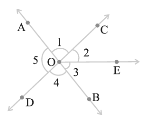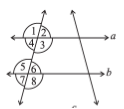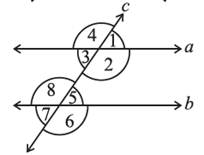### Lines and Angles - Worksheets

CBSE Worksheet-1

CLASS – VII Mathematics (Lines and Angles)

Choose correct option in questions 1 to 4.

1. How many points a line segment have?
a. 2
b. 1
c. 3
d. 0
2. In the following figure which angle is adjacent to ∠1?a. ∠3
b. ∠2
c. ∠5
d. both b and c
3. If a line is a transversal to three lines, how many points of intersections are there?
a. 1
b. 2
c. 3
d. 4
4. State the property that is used below: If || b, then ∠1 = ∠5.a. alternate interior angles
b. pair of interior angle
c. vertically opposite angles
d. corresponding angles

Fill in the blanks:

1. When the sum of the measures of two angles is 90°, the angles are called
___________.
2. _________ angles have a common vertex and a common arm but no common interior points.
3. When two lines intersect, the vertically opposite angles so formed are _________.
4. Two lines and m intersect if they have a point in ________.
5. When a transversal cuts two lines, such that pairs of corresponding angles are equal, then the lines have to be _______.
6. In the following figure, identify the pairs of corresponding angles.CBSE Worksheet-1
CLASS – VII Mathematics (Lines and Angles)